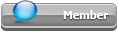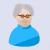## Featured Articles

Check out the latest featured articles.## File Library0# Fenske Equation Understanding And Application

2 replies to this topic
|

### #1Kiran T

Kiran T

Junior Member

•• Members
•• 23 posts

Posted 25 February 2019 - 06:46 AM

Dear Experts,

For binary mixtures, min number of stage can be calculated using fenske equation.

My question is , can i use weight fraction instead of mole fraction in this equation ?

this question is comes into the picture because sum of weight fraction and mole fraction will be 1 in binary mixture.

Regards!

Kiran

### #2Pilesar

Pilesar

Gold Member

•• Members
•• 574 posts

Posted 25 February 2019 - 07:23 AM

Your question is easy enough to test. If you do not calculate the exact same stages, then you will know for sure.

### #3imeagor

imeagor

Brand New Member

•• Members
•• 6 posts

Posted 18 April 2019 - 03:05 AM

really simple, just do some mathematical derivation and then you will know

### Similar Topics